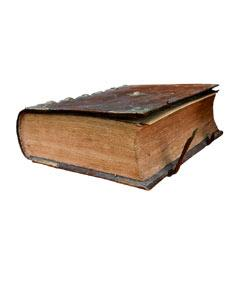# Shelving Book Test 3

8 QuestionsSettingsThe shelving test will be scored using the following criteria: You have 25 minutes to complete the test, with a minimum score of 75%. You will only be considered for a position if the above criteria is met. When arranging materials, sort first by the whole number (three digits before the decimal) and then, if there is more than one item with the same whole number, by the number following the decimal point. For example: 800 808. 09 808. 1 808. 14 808. 2 808. 321 Keep in mind that the number after the decimal point is a fraction, so that in the example above. 14 is smaller than. 2, so the item with the number 808. 14 comes before the one with the number 808. 2 Books classed as fiction do not have call numbers. They are arranged on the shelves alphabetically by the last name of the author, i